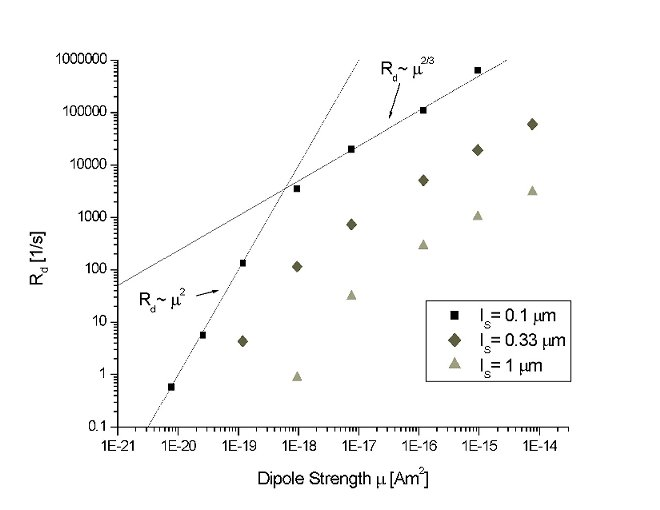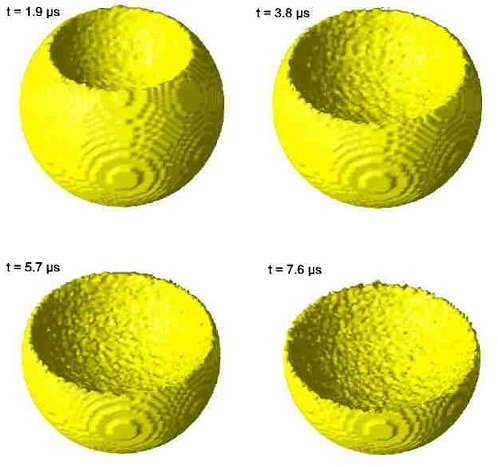Hahn spin echoes in porous media with large internal fields

NMR dephasing behavior of the nuclear spins of a fluid confined in a porous material can be investigated by Hahn spin echoes. Previous experimental results on water in a magnetically doped clay have shown a non-mono exponentially decaying magnetization, which can be understood neither by the known dephasing rate of freely diffusing spins in a uniform gradient nor by spins diffusing in a restricted geometry. For a better understanding of NMR measurements on these systems, a systematic survey was performed of the various length scales that are involved. The standard length scales for the situation of a uniform gradient are diffusing length, structural length and dephasing length. We show that for a non-uniform gradient, a new length scale has to be introduced: the magnetic-field curvature length. When a particle diffuses less than this length scale, it experiences a local uniform gradient. In that case the so-called Local Gradient Approximation (LGA) can describe the spin-echo decay. When a particle diffuses over a longer distance than the structure length, the spin-echo decay can be described by the motional averaging regime. For both regimes, scaling laws are derived. We have used a random-walk model to simulate the dephasing effect of diffusing spins in a spherical pore in the presence of a magnetic dipole field. By varying the dipole magnitude, situations can be created in which the dephasing behaviour scales according to the motional averaging regime or according to the LGA regime, for certain ranges of echo times. Two model systems are investigated: a spherical pore in the vicinity of a magnetic point dipole and a spherical pore adjacent to a magnetic dipolar grain of the same size as the pore. The simulated magnetization decay curves of both model systems confirm the scaling laws, see figure 1.Figure 1 – The dephasing rate Rd as a function of the magnetic dipole strength  for three pore sizes.
The solid lines represent the scaling laws for the motional averaging regime (slope=2) and the LGA situation (slope=2/3).

The LGA, characterized by a non-mono-exponential magnetization decay, is also investigated by calculating the spatially resolved magnetization in the pore, see figure 2. For this regime, the magnetization is found to be inhomogeneously distributed within the pore, whereas it is homogeneously distributed in the motional averaging regime.Figure 2 – The iso-magnetization surface M0 /2 for four successive spin-echo times.
The magnetic dipole (m =10^-14 Am^2) is situated at the top of the pore with a radius of 3 mm.

• An extensive description can be found in:
• R.M.E. Valckenborg, H.P. Huinink, J.J. v.d. Sande, and K. Kopinga, Random walk simulations of NMR dephasing effects due to uniform magnetic field gradients in a pore, Phys. Rev. E. 65, 021306 (2002)

R.M.E. Valckenborg, H.P. Huinink and K. Kopinga; NMR Dephasing Effects due to a magnetic dipole in a spherical pore, Journal of Chemical Physics 118, 3243-3251 (2003)